# Basic College Mathematics

Mathematics

## Quiz 11 : AppLooking for Calculus Homework Help?

## Quiz 11 :App

Question TypeFind the next number in the sequence. Describe the pattern in the sequence. See Examples. 2, 9, 16, 23, 30,…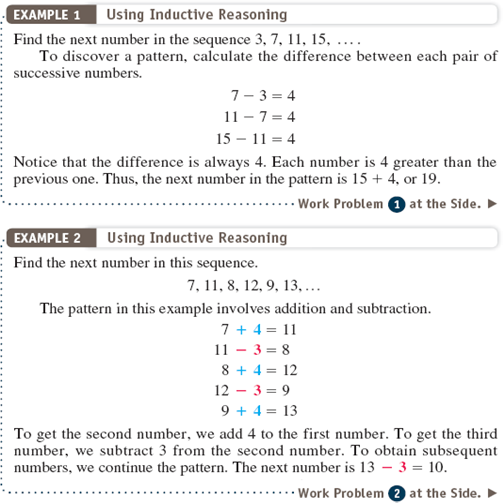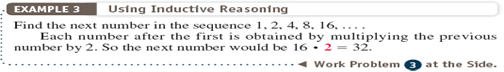Free
Essay

We wish to find here the next number in sequence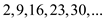To discover a pattern, calculate the difference between each pair of successive numbers.The difference is always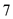. Each number isgreater than the previous one. Thus the next number of the pattern is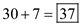TagsFind the next number in the sequence. Describe the pattern in the sequence. See Examples. 5, 8, 11, 14, 17,…Free
Essay

We wish to find here the next number in sequence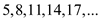To discover a pattern, calculate the difference between each pair of successive numbers.The difference is always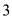. Each number isgreater than the previous one. Thus the next number of the pattern is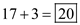TagsFind the next number in the sequence. Describe the pattern in the sequence. See Examples. 0, 10, 8, 18, 16,…Free
Essay

We wish to find here the next number in sequence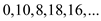The pattern in the above sequence involves addition and subtractionTo get the second number, we add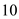to the first number. To get the third number, we subtract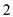from the second number. Thus the next number of the pattern is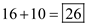TagsFind the next number in the sequence. Describe the pattern in the sequence. See Examples. 1, 4, 16, 64,…Essay
TagsFind the next number in the sequence. Describe the pattern in the sequence. See Examples. 1, 3, 9, 27, 81,…Essay
TagsFind the next number in the sequence. Describe the pattern in the sequence. See Examples. 3, 6, 12, 24, 48,…Essay
TagsFind the next number in the sequence. Describe the pattern in the sequence. See Examples 1, 4, 9, 16, 25, …Essay
TagsFind the next number in the sequence. Describe the pattern in the sequence. See Examples. 6, 7, 9, 12, 16,…Essay
TagsFind the next shape in the sequence. See Example 4.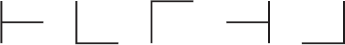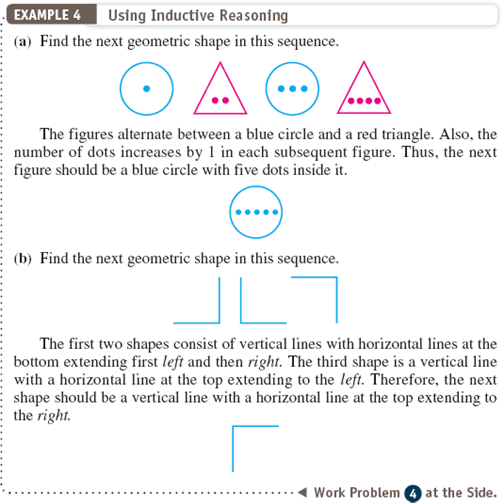Essay
TagsFind the next shape in the sequence. See Example 4.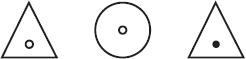Essay
TagsFind the next shape in the sequence. See Example 4.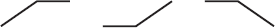Essay
TagsFind the next shape in the sequence. See Example 4.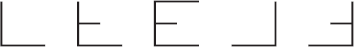Essay
TagsFor each argument, draw Euler circles and then state whether or not the conclusionfollows from the premises. See Examples All animals are wild. All lions are animals. All lions are wild.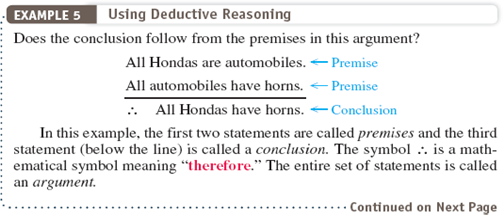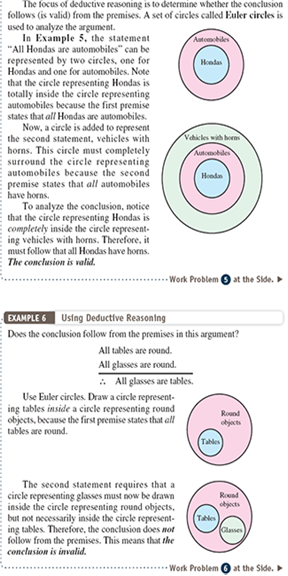Essay
TagsFor each argument, draw Euler circles and then state whether or not the conclusionfollows from the premises. See Examples All students are hard workers. All business majors are students. All business majors are hard workers.Essay
TagsFor each argument, draw Euler circles and then state whether or not the conclusionfollows from the premises. See Examples All teachers are serious. All mathematicians are serious. All mathematicians are teachers.Essay
TagsFor each argument, draw Euler circles and then state whether or not the conclusionfollows from the premises. See Examples All boys ride bikes. All Americans ride bikes. All Americans are boys.Essay
TagsSolve the application problem. See Examples In a given 30-day period, a husband watched television 20 days and his wife watched television 25 days. If they watched television together 18 days, how many days did neither watch television Draw a Venn diagram.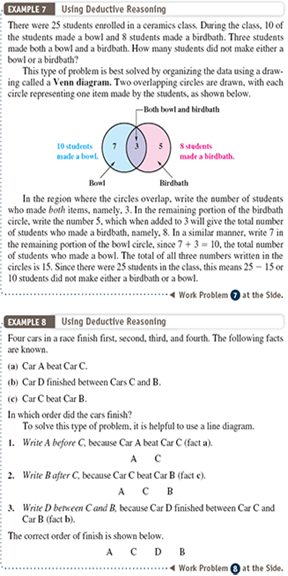Essay
TagsSolve the application problem. See Examples In a class of 40 students, 21 students take both calculus and physics. If 30 students take calculus and 25 students take physics, how many do not take either calculus or physics Draw a Venn diagram.EssaySolve the application problem. See Examples Tom, Dick, Mary, and Joan all work for the same company. One is a secretary, one is a computer operator, one is a receptionist, and one is a mail clerk. (a) Tom and Joan eat dinner with the computer operator. (b) Dick and Mary carpool with the secretary. (c) Mary works on the same floor as the computer operator and the mail clerk. Who is the computer operatorSolve the application problem. See Examples Four cars-a Ford, a Buick, a Mercedes, and an Audi-are parked in a garage in four spaces. (a) The Ford is in the last space. (b) The Buick and Mercedes are next to each other. (c) The Audi is next to the Ford but not next to the Buick. Which car is in the first space